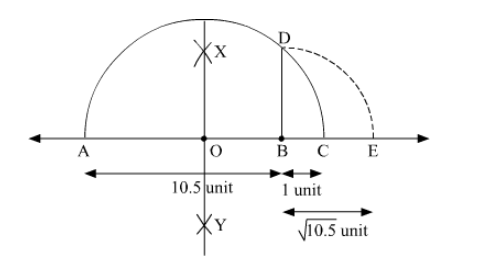Deepak Scored 45->99%ile with Bounce Back Crack Course. You can do it too!

# Represent 10.5−−−−√ on the number line.

Question:

Represent $\sqrt{10.15}$ on the number line

Solution:To represent $\sqrt{10.5}$ on the number line, follow the following steps of construction:

(i) Mark two points A and B on a given line such that AB = 10.5 units.

(ii) From B, mark a point C on the same given line such that BC = 1 unit.

(iii) Find the mid point of AC and mark it as O.

(iv) With O as centre and radius OC, draw a semi-circle touching the given line at points A and C.

(v) At point B, draw a line perpendicular to AC intersecting the semi-circle at point D.

(vi)  With B as centre and radius BD, draw an arc intersecting the given line at point E.

Thus, let us treat the given line as the number line, with $B$ as $0, C$ as 1 , and so on, then point $E$ represents $\sqrt{10.5}$.

Justification:

Here, in semi-circle, radii $\mathrm{OA}=\mathrm{OC}=\mathrm{OD}=\frac{10.5+1}{2}=\frac{11.5}{2}=5.75$ units

And, $\mathrm{OB}=\mathrm{AB}-\mathrm{AO}=10.5-5.75=4.75$ units

In a right angled triangle OBD,

$\mathrm{BD}=\sqrt{\mathrm{OD}^{2}-\mathrm{OB}^{2}}$

$=\sqrt{5.75^{2}-4.75^{2}}$

$=\sqrt{(5.75+4.75)(5.75-4.75)} \quad\left[\mathrm{a}^{2}-\mathrm{b}^{2}=(\mathrm{a}+\mathrm{b})(\mathrm{a}-\mathrm{b})\right]$

$=\sqrt{10.5 \times 1}$

$=\sqrt{10.5}$